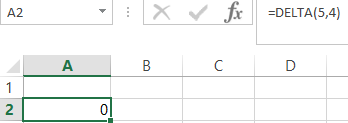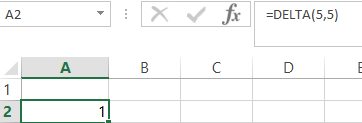## Engineering Functions

The built-in Excel Engineering Functions have been provided to perform some of the commonly used engineering calculations. In this article we are going to learn how to use EXCEL engineering function.

CONVERT Function

The CONVERT function is used to convert a value from one type to another. For example meter to yard.CONVERT function is:

=CONVERT (number, from_unit, to_unit)

• number – the number you want to convert,
• from_unit – the current unit
• to_unit – the unit you want the value to convert in.

Example:

=Convert (2000,”m”,”yd”)

Explanation: This will convert the value 2000 from meter to yards.IMSUM Function

Returns the sum of a complex numbers.

The syntax of the IMSUM function is:

=IMSUM (inumber1, inumber2…)

• inumber1 and inumber2 – complex numbers from 1 to 29 to add.

Example:

=IMSUM(“3+4i”, “5-3i”)IMREAL Function

Returns the real coefficient of a complex number in x + yi or x + yj text format

The Syntax of the IMREAL Function is:

=IMREAL (inumber)

• Inumber – a complex number for which you want the real coefficient.Explaination: The real coefficient of 6-9i is 6.

DELTA Function

Tests whether two values are equal. Returns 1 if number1 = number2; returns 0 otherwise

The Syntax of the DELTA Function is:

=DELTA (number1, number2)

• Number1 is the first number and Number2 is the second number. If omitted, number2 is assumed to be zero.

Examples:Explanation: Number 5 is not equal to number 4 so the result is 0.Explanation: Number 5 is equal to number 5 so the result is 1.

ERF Function

Returns the error function integrated between lower_limit and upper_limit.

The syntax of the ERF Function is:

=ERF(lower_limit, upper_limit)

• Lower_limit is the lower bound for integrating ERF
• Upper_limit is the upper bound for integrating ERF. If omitted, ERF integrates between zero and lower_limit.

Example:Explanation: Error function integrated between 0 and 0.745000 is 0.707929

## Template

```Further reading: